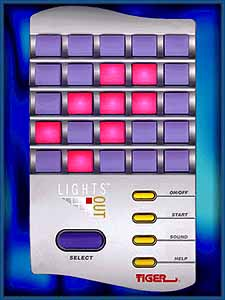# The Lights Out Game

In this short post, I will discuss a cute graph theory problem called the Lights Out Game. The set-up is as follows.  We are given a graph $G=(V,E)$, where we consider each vertex as a light.  At the beginning of the game, some subset of the vertices are ON, and the rest of the vertices are OFF.  We are allowed to press any vertex $v$, which has the effect of switching the state of $v$ and all of the neighbours of $v$.  The goal is to determine if it is possible to press some sequence of vertices to turn off all the lights.

The version of this game where $G$ is the $5 \times 5$ grid was produced in the real world by Tiger Electronics, and has a bit of a cult following.  For example, there is a dedicated wikipedia page and this site (from which I borrowed the image below) even has the original user manuals.The goal of this post is to prove the following theorem.

Theorem. Let $G=(V,E)$ be a graph for which all the lights are initially ON.  Then it is always possible to press a sequence of vertices to switch all the lights OFF.

(Note that if not all the lights are ON initially, it will not always be possible to switch all the lights OFF.  For example, consider just a single edge where only one light is ON initially.)

Proof. Evidently, there is no point in pressing a vertex more than once, and the sequence in which we press vertices does not matter.  Thus, we are searching for a subset $S$ of vertices such that $|S \cap N[v]|$ is odd for all $v \in V$, where $N[v]$ is the closed neighbourhood of $v$ (the neighbours of $v$ together with $v$ itself).  We can encode the existence of such a set $S$ by doing linear algebra over the binary field $\mathbb{F}_2$.

Let $A$ be the $V \times V$ adjacency matrix of $G$, and let $B=A+I$. Note that the column corresponding to a vertex $v$ is the characteristic vector of the closed neighbourhood of $v$. Thus, the required set $S$ exists if and only if the all ones vector $\mathbb{1}$ is in the column space of $B$.  Since $B$ is symmetric, this is true if and only if $\mathbb{1}$ is in the row space of $B$.  Let $B^+$ be the matrix obtained from $B$ by adjoining $\mathbb{1}$ as an additional row. Thus, we can turn all the lights OFF if and only if $B$ and $B^+$ have the same rank.

Since this is a matroid blog, let $M(B)$ and $M(B^+)$ be the column matroids of $B$ and $B^+$ (over $\mathbb{F}_2$). We will prove the stronger result that $M(B)$ and $M(B^+)$ are actually the same matroid. Every circuit of $M(B^+)$ is clearly a dependent set in $M(B)$.  On the other hand let $C \subseteq V$ be a circuit of $M(B)$.  Then $\sum_{v \in C} \chi(N[v])=\mathbb{0}$, where $\chi(N[v])$ is the characteristic vector of the closed neighbourhood of $v$. Therefore, in the subgraph of $G$ induced by the vertices in $C$, each vertex has odd degree.  By the Handshaking Lemma, it follows that $|C|$ is even, and so $C$ is also dependent in $M(B^+)$.

Reference

Anderson, M., & Feil, T. (1998). Turning lights out with linear algebra. Mathematics Magazine, 71(4), 300-303.

This site uses Akismet to reduce spam. Learn how your comment data is processed.